In this chapter, you will find numbers that make statements true. This is called solution of equations. You will solve equations in two different ways, by inspection and by â€˜reversingâ€™ them. You will find that two equations can have the same solution. Such equations are called equivalent equations. You will also discover that not all statements are algebraic equations. Some statements are algebraic identities and others are in fact algebraic impossibilities. You will learn what the difference is between these three types of statements.

## Solving equations by inspection

1. Six equations are listed below the table. Use the table to find out for which of the given values of $x$ it will be true that the left-hand side of the equation is equal to the right-hand side.

"Searching" for the solution of an equation by using tables is called solution by inspection.

 $x$ -3 -2 -1 0 1 2 3 4 $2x + 3$ -3 -1 1 3 5 7 9 11 $x + 4$ 1 2 3 4 5 6 7 8 $9 - x$ 12 11 10 9 8 7 6 5 $3x- 2$ -11 -8 -5 -2 1 4 7 10 $10x - 7$ -37 -27 -17 -7 3 13 23 33 $5x+ 3$ -12 -7 -2 3 8 13 18 23 $10 - 3x$ 19 16 13 10 7 4 1 -2
1. $2x+ 3 = 5x + 3$

2. $5x + 3 = 9 -x$

3. $2x+ 3 =x + 4$

4. $10x- 7 = 5x + 3$

5. $3x - 2 = x + 4$

6. $9 - x = 2x + 3$

Two equations can have the same solution. For example, $5x= 10$ and $x + 2 = 4$ have the same solution; $x= 2$ is the solution for both equations.

Two equations are called equivalent if they have the same solution.

1. Which of the equations in question 1 have the same solutions? Explain.

## Solving equations using additive and multiplicative inverses

1. In each case find the value of $x$:

1.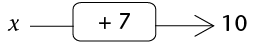2.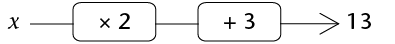2. Complete the flow diagrams. You have to fill in all the missing numbers.

To find the second input number you may say to yourself, "After I added 7, I had 12. What did I have before I added 7?"

1.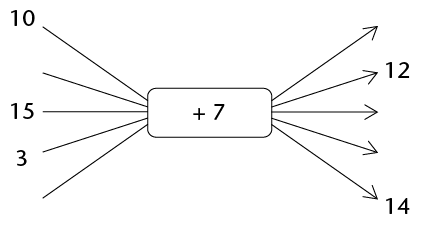To find the input number that corresponds to 13, you may ask yourself, "What did I have before I added 3?" and then, "What did I have before I multiplied by 2?"

2.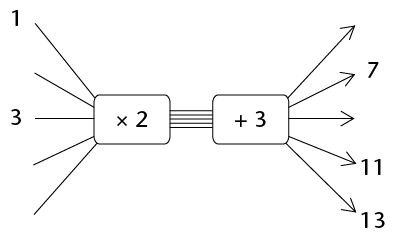4. Describe the instructions in flow diagram 2(b) in words, and also with a symbolic expression.

5. Complete the flow diagram.

This flow diagram is called the inverse of the flow diagram in question 2(b).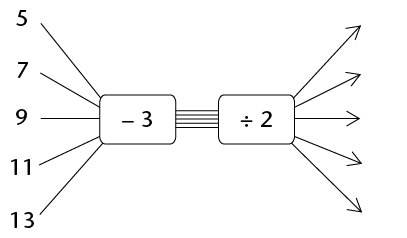6. Compare the input numbers and the output numbers of the flow diagrams in question 2(b) and question 5. What do you notice?

1. Add 5 to any number and then subtract 5 from your answer. What do you get?

2. Multiply any number by 10 and then divide the answer by 10. What do you get?

If you add a number and then subtract the same number, you are back where you started. This is why addition and subtraction are called inverse operations. If you multiply by a number and then divide by the same number, you are back where you started. This is why multiplication and division are called inverse operations

The expression $5x-3$ says "multiply by 5 then subtract 3". This instruction can also be given with a flow diagram: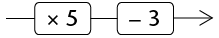The equation $5x - 3 = 47$ can also be written as a flow diagram: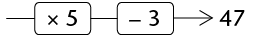7. Solve the equations below. You may do this by using the inverse operations. You may write a flow diagram to help you to see the operations.

1. $2x + 5 = 23$

2. $3x - 5 = 16$

3. $5x - 60 = -5$

4. $\frac{1}{3}x + 11 = 19$

5. $10(x+ 3) = 88$

6. $2(x - 13) = 14$

## Setting up equations

### Constructing equations

You can easily make an equation that has 5 as the solution. Here is an example:

 Start by writing the solution $x = 5$ Add 3 to both sides $x +3 = 8$ Multiply both sides by 5 $5x + 15 = 40$
1. What is the solution of the equation $5x + 15 = 40$?

2. Make your own equation with the solution $x= 3$.

3. Bongile worked like this to make the equation $2(x$ + 8) = 30, but he rubbed out part of his work:

 Start by writing the solution $x$ = Add 8 to both sides = 15 Multiply both sides by 2 $2(x + 8)$ = 30

Complete Bongile's writing to solve the equation $2(x + 8) = 30$.

4. This is how Bongile made a more difficult equation:

 Start by writing the solution $x$ = Multiply by 3 on both sides $3x$ = Subtract 9 from both sides $3x - 9$ = 6 Add $2x$ to both sides $5x - 9$ = $2x + 6$
1. What was on the right-hand side before Bongile subtracted 9?

2. What is the solution of $5x - 9 = 2x + 6$?

5. Bongile started with a solution and he ended up with an equation. Fill in the steps that Bongile took to make the equation, and solve the equation:

 $x$ = $8x$ = $8x+ 3$ = $3x+ 3$ = $35 - 5x$

### Solving equations

 To make an equation, you can apply the same operation on both sides To solve an equation, you can apply the inverse operation on both sides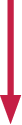$x$ = 4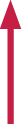Multiply by 8 $8x$ = 32 Divide by 8 Add 3 $8x+ 3$ = 35 Subtract 3 Subtract $5x$ $3x+ 3$ = $35 - 5x$ Add $5x$

Use any appropriate method to solve the equations below.

1. $5x + 3 = 24 - 2x$

2. $2x+ 4 = -9$

3. $3 - x = x- 3$

4. $6(2x + 1) = 0$

1. $4(1 - 2x) = 12 - 7x$

2. $8(1 - 3x) = 5(4x + 6)$

3. $7x- 10 = 3x + 7$

4. $1,6x + 7 = 3, 5x+ 3,2$

### Number patterns and equations

1. Which of the following rules will produce the number pattern given in the second row of the table below?

A. Term value = 8n where n is the term number

B. Term value = 6n - 1 where n is the term number

C. Term value = 6n + 2 where n is the term number

D. Term value = 10n - 2 where n is the term number

E. Term value = 5n + 3 where n is the term number

 Term number 1 2 3 4 5 6 7 8 9 Term value 8 13 18 23 28 33 38 43 48
2. The sixth term of the sequence has the value 33. Which term will have the value 143? You may set up and solve an equation to find out.

1. Write the rule that will produce the number pattern in the second row of this table. You may have to experiment to find out what the rule is.

 Term number 1 2 3 4 5 6 7 8 9 Term value 5 8 11 14 17 20 23 26 29

2. Which term will have the value 221?

1. The rule for number pattern A is 4n + 11, and the rule for pattern B is $7n - 34$.

1. Complete the table below for the two patterns.

 Term number 1 2 3 4 5 6 7 8 9 Pattern A Pattern B
2. For which value of n are the terms of the two patterns equal?

## Equation and situations

1. Consider this situation:

To rent a room in a certain building, you have to pay a deposit of R400 and then R80 per day.

1. How much money do you need to rent the room for 10 days?

2. How much money do you need to rent the room for 15 days?

2. Which of the following best describes the method that you used to do question 1(a) and (b)?

A. Total cost = R400 + R80

B. Total cost = 400(number of days + 80)

C. Total cost = 80 $\times$ number of days + 400

D. Total cost = (80 + 400) $\times$ number of days

3. For how many days can you rent the room described in question 1, if you have R2 800 to pay?

If you want to know for how many days you can rent the room if you have R720, you can set up an equation and solve it:

You know the total cost is R720 and you know that you can work out the total cost like this:

$\text{Total cost} = 80x + 400$, where $x$ is the number of days. So, $80x + 400 = 720$ and $x = 4$ days.

In each of the following cases, find the unknown number by setting up an equation and solving it.

4. To rent a certain room, you have to pay a deposit of R300 and then R120 per day.

1. For how many days can you rent the room if you can pay a total of R1 740? (If you experience trouble in setting up the equation, it may help you to decide first how you will work out what it will cost to rent the room for 6 days.)

2. What will it cost to rent the room for 10 days, 11 days and 12 days?

3. For how many days can you rent the room if you have R3 300 available?

4. For how many days can you rent the room if you have R3 000 available?

5. Ben and Thabo decide to do some calculations with a certain number. Ben multiplies the number by 5 and adds 12. Thabo gets the same answer as Ben when he multiplies the number by 9 and subtracts 16. What is the number they worked with?

6. The cost of renting a certain car for a period of $x$ days can be calculated with the following formula:

$\text{Rental cost in rand} = 260x + 310$

What information about renting this car will you get, if you solve the equation

$260x+ 310 = 2 910$?

7. Sarah paid a deposit of R320 for a stall at a market, and she also pays R70 per day rental for the stall. She sells fruit and vegetables at the stall, and finds that she makes about R150 profit each day. After how many days will she have earned as much as she has paid for the stall, in total?

## Solving equations by using the laws of exponents

You may need to look back at Chapter 5 to remember the laws of exponents.

One kind of exponential equation that you deal with in Grade 9 has one or more terms with a base that is raised to a power containing a variable.

Example: $2^{x} = 16$

When we need to find the unknown value, we are asking the question: "To what power must the base be raised for the statement to be true?"

Example: $2^{x}= 16$ Make sure that the terms with $x$ are on their own on one side.

$2^{x} = 2^{4}$ Write the known term in the same base as the term with the exponent.

$x= 4$ Equate the exponents.

In the example above, we can equate the exponents because the two numbers are equal only when they are raised to the same power.

1. Solve for $x$:

1. $5^{x-1} = 125$

2. $2^{x+3}= 8$

3. $10^{x}= 10 000$

4. $4^{x+2}= 64$

5. $7^{x+1}= 1$

6. $x^{0}= 1$

Example: Solve for $x$: $3^{x} = \frac{1}{27}$

$3^{x} = 3^{-3}$ (Rewrite $\frac{1}{27}$ as a number to base 3)

$x = -3$ (Equate the exponents.)

2. Solve for $x$.

1. $7^{x} = \frac{1}{49}$

2. $10^{x}= 0,001$

3. $6^{x} = \frac{1}{216}$

4. $10^{x-1}= 0,001$

5. $4^{-x} = \frac{1}{16}$

6. $7^{x} = 7^{-3}$

In another kind of equation involving exponents, the variable is in the base.

When we need to find the unknown value, we are asking the question: "Which number must be raised to the given power for the statement to be true?"

For these equations, you should remember what you know about the powers of numbers such as 2, 3, 4, 5 and 10.

### Solving equations with a variable in the base

1. Complete the table below and answer the questions that follow:

 $x$ 2 3 4 5 (a) $x^{3}$ $2^{3}= 8$ (b) $x^{5}$ $2^{5}= 32$ (c) $x^{4}$ $2^{4}= 16$

For what value of $x$ is:

1. $x^{3}= 64$

2. $x^{5}= 32$

3. $x^{4}= 256$

4. $x^{3}= 8$

5. $x^{4}= 16$

6. $x^{5}= 3 125$

2. Solve for $x$ and give a reason:

1. $x^{3}= 216$

2. $x^{2}= 324$

3. $x^{4}= 10 000$

4. $8^{x} = 512$

5. $18^{x}= 324$

6. $6^{x}= 216$

1. Ahmed multiplied a number by 5, added 3 to the answer, and then subtracted the number he started with. The answer was 11. What number did he start with?

2. Use any appropriate method to solve the equations.

1. $3(x-2) = 4(x+1)$

2. $5(x+2) = -3(2-x)$

3. $1,5x = 0,7x âˆ’ 24$

4. $5(x + 3) = 5x + 12$
5. $2,5x = 0,5(x + 10)$

6. $7(x âˆ’ 2) = 7(2 âˆ’ x)$

7. $\frac{1}{2}(2x-3)=5$

8. $2x âˆ’ 3(3 + x) = 5x + 9$